• Save

# Calendar Problems Formula for Competitive Exams

7 months ago 2.0K Views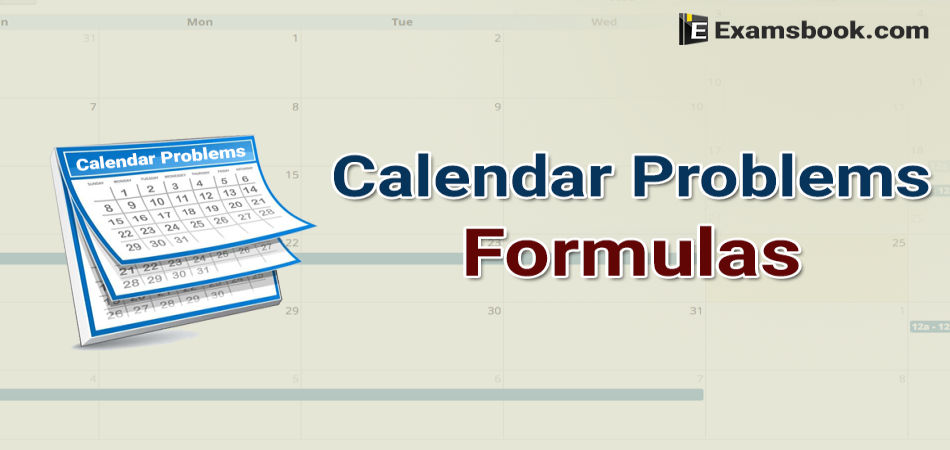Most of the students are facing problems while solving calendar questions in the competitive exam. If you don't want to face the same problem while solving calendar questions in the exam, you should practice with given calendar problems formulas.

You can easily obtain good marks in the competitive exam if you prepare one-two hour's daily these calendar problems formula. Here I have explained to this topic in detail and as well as solved calendar problems also.

## Calendar Problems with Using Formula

### What is Calendar ?

Calendar measure a day, a week, a month and a year. In other words, we can say that calendar is the measurement of a year.

Question based on calendar are of set pattern and hence follow set rules. We are giving below the definition/meaning of certain words which are used in solving question on calendar.

Odd Days: we know that a week contains 7 days counting from Monday to Sunday. So, any number of days, which are more than complete number of a week in a given period are called odd days. E.g., a period of 10 days contains 3 odd days, 11 days contains 4 odd days, 12 days contains 5 odd days. But period of 14 days contains zero odd day.

Therefore, In finding number of odd days in a given period of time, one has to divide that period by 7. If it is completely divisible by 7, it contains zero odd day and if it is not divisible by 7, then remaining number of days are the odd days.

Leap Year: The Year which is divisible is by 4 is called a leap year. But every century which is divisible by 4 is not a leap year. Every 4th century is a leap year. E.g., 400, 800, 1200, 1600,……are all leap years but centuries like 100, 300, 500, 600,………are not leap years.

An ordinary year has 365 days i.e, (52 weeks + 1 day)

A leap year has 366 days i.e (52 weeks + 2 days)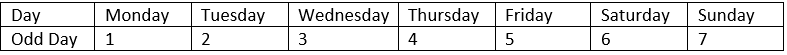### Important Formula and Tips

1. Normally an year contain 365 days. i.e. 52 weeks and a day.

2. But a leap year contains 366 days i.e. 52 weeks and two days because, February of a leap year contains 29 days.

3. If an year is exactly divided by 4, it is a leap year.

4. In an year January, March, May, July, August, October and December contain 31 days. While, April, June, September and November contain 30 days.

5. After 7 days i.e., after a week a day repeats itself. E.g. If 11th July is Thursday, then 18th July will also be Thursday.

6. In 100 yr contains 76 simple years, 24 leap and 5 odd days.

7. In 200 yr =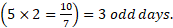8.  300 yr =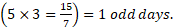9.  400 yr =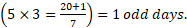10. In a simple year, months that have same first day January – October

February – March – November April – July

11. In a leap year, months that have same first day

January – April – July  February – August    March – November

12. First and the last day of a simple year remain same as 1st January is on Monday. So  the 31st December of that year will be on Monday as well.

13. Last day of a leap year is increased by one day with reference to the first of that year. As 1st January of a leap year is on Monday. So the 31st December will be on Tuesday as well.

Example: 1. What was the day on 1st January, 1901?

(A) Monday

(B) Wednesday

(C) Sunday

(D) Tuesday

Solution:

1st January, 1901 means (1900 yr and 1 day)

Now, 1600 yr have 0 odd day

300 yr have 1 odd day

1 day has 1 odd day

Total number of odd days

= 0+1+1 = 2 days.

Example : 2. What is the day on 31st October, 1984?

(A) Friday

(B) Thursday

(C) Wednesday

(D) Monday

Solution:

31st October 1984 means

(1983 yr and 10 month)

Now, 1600 yr have 0 odd days

300 yr have 1 odd day

83 yr have 20 leap year and 63 ordinary years

= (40+63) odd days

= (103) odd days i.e. 5 odd days

10 month of the year 1984 have 305 days.

(February is of 29 days, being the month of leap year)

305 days have (43 weeks and 4 days) or 4 odd days.

Total number of odd days = (1+5+4) = 10 odd days or 3 odd days

Hence, it was Wednesday on 31st October, 1984.

Example: 3. What was the day on 14th March, 1993?

(A) Friday

(B) Thursday

(C) Sunday

(D) Saturday

Solution:

14th march, 1993 means (1992 yr,

2 months and 14 days)

Now, 1990 yr have 1 odd day

92 yr have 23 leap year and 69 ordinary years = (46+69)

= 115 days = 3 odd days

2 months + 14 days

= 31+28+14=73=3 odd days.

Total number of odd days = (1+3+3) = 7 odd days.

Hence, it was Sunday on 14th March 1993.

Example 4. Today is Wednesday, what will be the day after 94 days?

(A) Monday

(B) Tuesday

(C) Wednesday

(D) Sunday

Solution:

Every day of the week is repeated after 7 days. Hence It will be Wednesday after 94 days.

Example 5. Today is Thursday. The day after 59 days will be

(A) Sunday

(B) Monday

(C) Tuesday

(D) Wednesday

Solution:

Every day of week is repeated after 7 days. Hence after 56 days, it would be Thursday again and after 59 days it would be Sunday.

You can ask me anything in the comment section regarding calendar problems formulas if you face any problem.